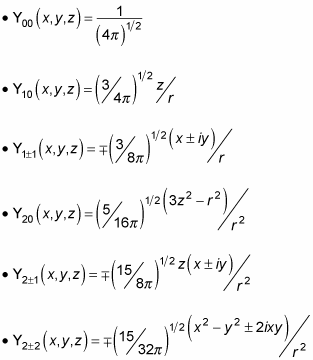##### Quantum Physics For Dummies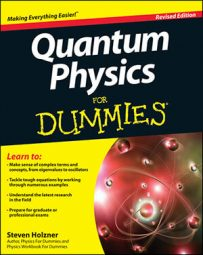Your quantum physics instructor may ask you to find the eigenfunctions of L2 in spherical coordinates. To do this, you start with the eigenfunction of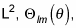given that in spherical coordinates, the L2 operator looks like this: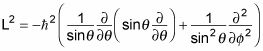That’s quite an operator. And, given that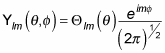you can apply the L2 operator to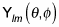which gives you the following: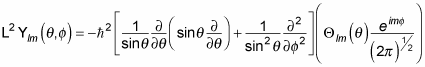And because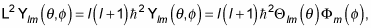this equation becomes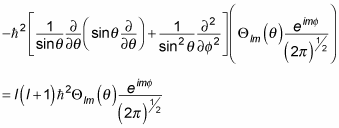Wow, what have you gotten into? Cancelling terms and subtracting the right-hand side from the left finally gives you this differential equation: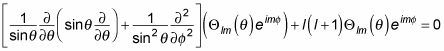Combining terms and dividing by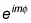gives you the following: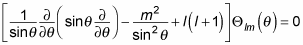Holy cow! Isn’t there someone who’s tried to solve this kind of differential equation before? Yes, there is. This equation is a Legendre differential equation, and the solutions are well known. (Whew!) In general, the solutions take this form: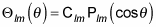where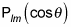is the Legendre function.

So what are the Legendre functions? You can start by separating out the m dependence, which works this way with the Legendre functions: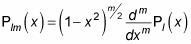where Pl(x) is called a Legendre polynomial and is given by the Rodrigues formula: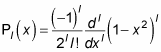You can use this equation to derive the first few Legendre polynomials like this: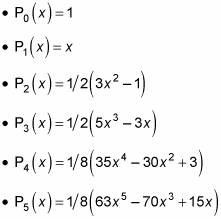and so on. That’s what the first few Pl (x) polynomials look like. So what do the associated Legendre functions, Plm (x) look like? You can also calculate them. You can start off with Pl0 (x), where m = 0. Those are easy because Pl0 (x) = Pl (x), so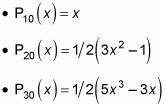Also, you can find that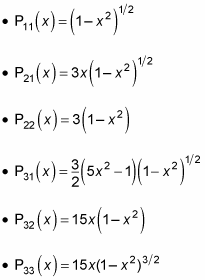These equations give you an overview of what the Plm functions look like, which means you’re almost done. As you may recall,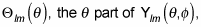is related to the Plm functions like this:And now you know what the Plm functions look like, but what do Clm, the constants, look like? As soon as you have those, you’ll have the complete angular momentum eigenfunctions,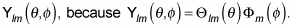You can go about calculating the constants Clm the way you always calculate such constants of integration in quantum physics — you normalize the eigenfunctions to 1.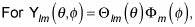that looks like this: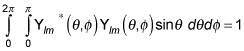(Remember that the asterisk symbol [*] means the complex conjugate. A complex conjugate flips the sign connecting the real and imaginary parts of a complex number.)

Substitute the following three quantities in this equation: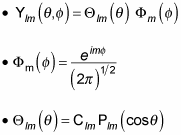You get the following: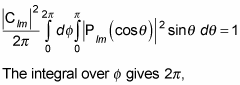so this becomes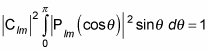You can evaluate the integral to this: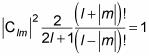So in other words: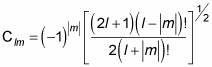Which means that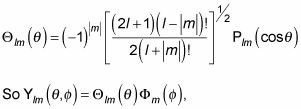which is the angular momentum eigenfunction in spherical coordinates, is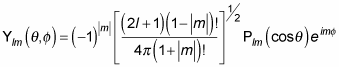The functions given by this equation are called the normalized spherical harmonics. Here are what the first few normalized spherical harmonics look like: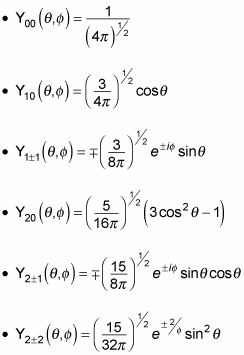In fact, you can use these relations to convert the spherical harmonics to rectangular coordinates: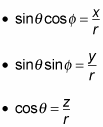Substituting these equations into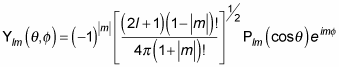gives you the spherical harmonics in rectangular coordinates: